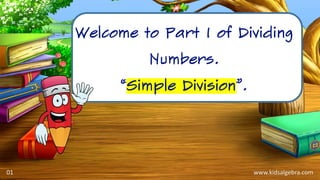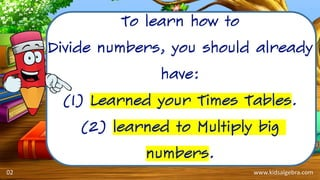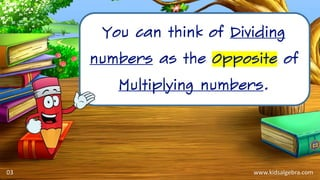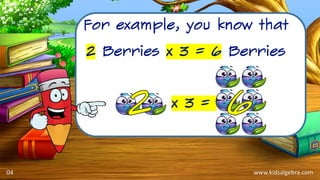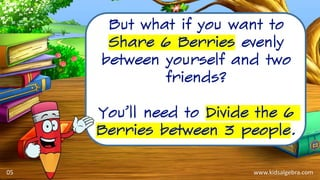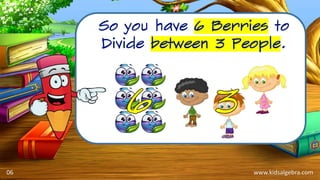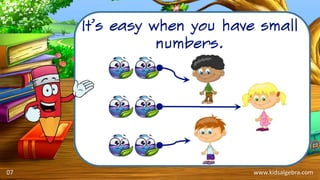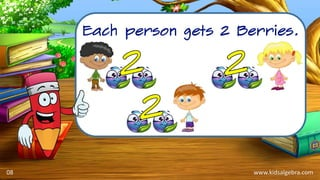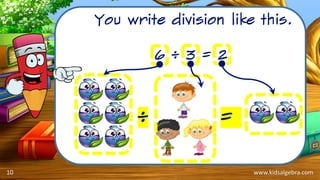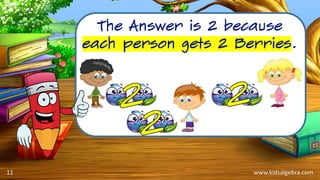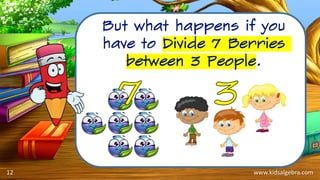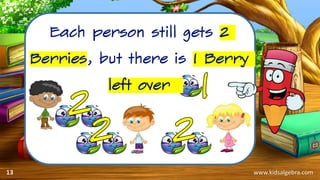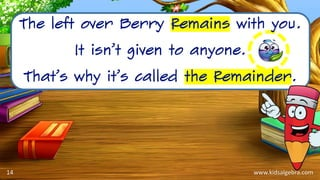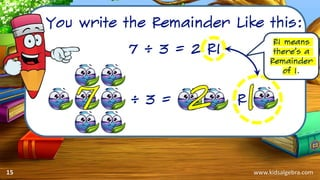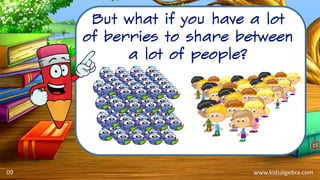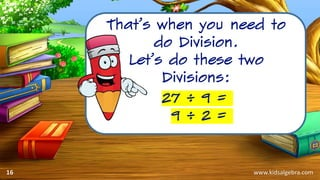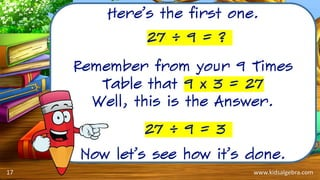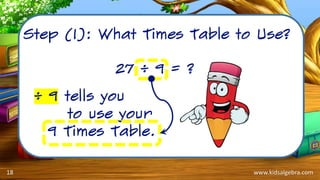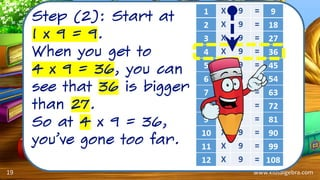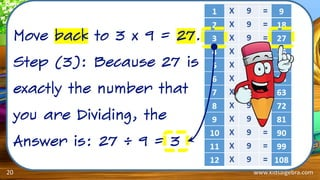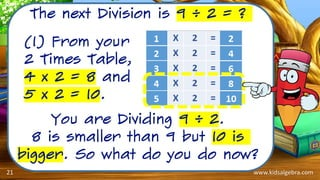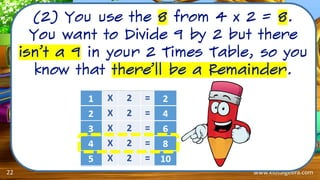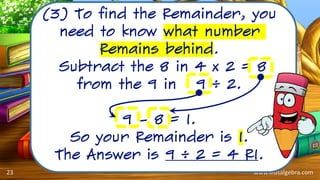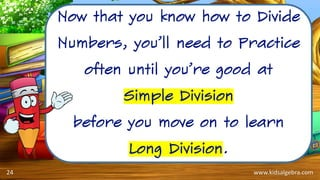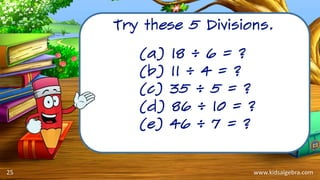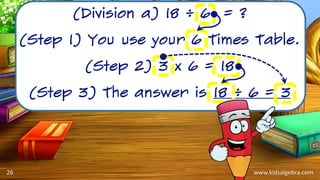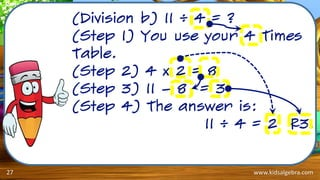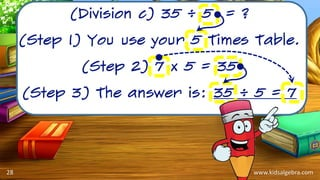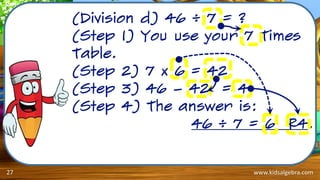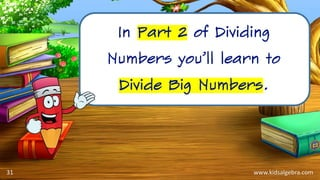1 of 31

### Simple Division for Primary School

1. www.kidsalgebra.com Welcome to Part 1 of Dividing Numbers. “Simple Division”. 01
2. www.kidsalgebra.com To learn how to Divide numbers, you should already have: (1) Learned your Times Tables. (2) learned to Multiply big numbers. 02
3. www.kidsalgebra.com You can think of Dividing numbers as the Opposite of Multiplying numbers. 03
4. www.kidsalgebra.com For example, you know that 2 Berries x 3 = 6 Berries x 3 = 04
5. www.kidsalgebra.com But what if you want to Share 6 Berries evenly between yourself and two friends? You’ll need to Divide the 6 Berries between 3 people. 05
6. www.kidsalgebra.com So you have 6 Berries to Divide between 3 People. 06
7. www.kidsalgebra.com It’s easy when you have small numbers. 07
8. www.kidsalgebra.com Each person gets 2 Berries. 08
9. www.kidsalgebra.com You write division like this. 6 ÷ 3 = 2. ÷ = 10
10. www.kidsalgebra.com The Answer is 2 because each person gets 2 Berries. 11
11. www.kidsalgebra.com But what happens if you have to Divide 7 Berries between 3 People. 12
12. www.kidsalgebra.com Each person still gets 2 Berries, but there is 1 Berry left over 13
13. www.kidsalgebra.com The left over Berry Remains with you. It isn’t given to anyone. That’s why it’s called the Remainder. 14
14. www.kidsalgebra.com You write the Remainder Like this: 7 ÷ 3 = 2 R1 ÷ 3 = R R1 means there’s a Remainder of 1. 15
15. www.kidsalgebra.com But what if you have a lot of berries to share between a lot of people? 09
16. www.kidsalgebra.com That’s when you need to do Division. Let’s do these two Divisions: 27 ÷ 9 = 9 ÷ 2 = 16
17. www.kidsalgebra.com Here’s the first one. Remember from your 9 Times Table that 9 x 3 = 27 Well, this is the Answer. Now let’s see how it’s done. 27 ÷ 9 = 3 27 ÷ 9 = ? 17
18. www.kidsalgebra.com Step (1): What Times Table to Use? 27 ÷ 9 = ? ÷ 9 tells you to use your 9 Times Table. 18
19. www.kidsalgebra.com Step (2): Start at 1 x 9 = 9. When you get to 4 x 9 = 36, you can see that 36 is bigger than 27. So at 4 x 9 = 36, you’ve gone too far. 19
20. www.kidsalgebra.com Move back to 3 x 9 = 27. Step (3): Because 27 is exactly the number that you are Dividing, the Answer is: 27 ÷ 9 = 3 20
21. www.kidsalgebra.com The next Division is 9 ÷ 2 = ? You are Dividing 9 ÷ 2. 8 is smaller than 9 but 10 is bigger. So what do you do now? (1) From your 2 Times Table, 4 x 2 = 8 and 5 x 2 = 10. 21
22. www.kidsalgebra.com (2) You use the 8 from 4 x 2 = 8. You want to Divide 9 by 2 but there isn’t a 9 in your 2 Times Table, so you know that there’ll be a Remainder. 22
23. www.kidsalgebra.com (3) To find the Remainder, you need to know what number Remains behind. Subtract the 8 in 4 x 2 = 8 from the 9 in 9 ÷ 2. 9 – 8 = 1. So your Remainder is 1. The Answer is 9 ÷ 2 = 4 R1. 23
24. www.kidsalgebra.com Now that you know how to Divide Numbers, you’ll need to Practice often until you’re good at Simple Division before you move on to learn Long Division. 24
25. www.kidsalgebra.com Try these 5 Divisions. 25 (a) 18 ÷ 6 = ? (b) 11 ÷ 4 = ? (c) 35 ÷ 5 = ? (d) 86 ÷ 10 = ? (e) 46 ÷ 7 = ?
26. www.kidsalgebra.com (Division a) 18 ÷ 6 = ? (Step 1) You use your 6 Times Table. (Step 2) 3 x 6 = 18 (Step 3) The answer is 18 ÷ 6 = 3 26
27. www.kidsalgebra.com (Division b) 11 ÷ 4 = ? (Step 1) You use your 4 Times Table. (Step 2) 4 x 2 = 8 (Step 3) 11 – 8 = 3 (Step 4) The answer is: 11 ÷ 4 = 2 R3. 27
28. www.kidsalgebra.com (Division c) 35 ÷ 5 = ? (Step 1) You use your 5 Times Table. (Step 2) 7 x 5 = 35 (Step 3) The answer is: 35 ÷ 5 = 7 28
29. www.kidsalgebra.com (Division d) 46 ÷ 7 = ? (Step 1) You use your 7 Times Table. (Step 2) 7 x 6 = 42 (Step 3) 46 – 42 = 4 (Step 4) The answer is: 46 ÷ 7 = 6 R4. 27
30. www.kidsalgebra.com In Part 2 of Dividing Numbers you’ll learn to Divide Big Numbers. 31
31. You can get a Wall Hanging at www.kidsalgebra.com to follow and practice Simple Division. www.kidsalgebra.com26